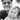# How to calculate Jordan's normal form (the hard way)

June 10, 2013

1. First thing we do is find out the spectrum of $A$, that is, its eigenvalues. We shall mark them as $\text{sp}A=\{\lambda_1, \lambda_2, \lambda_3, \cdots, \lambda_n\}$. We do this however we can, usually by finding the zeros of the characteristic polynomial, that is solving the equation $\det(A-\lambda I) = 0$ for $\lambda$
2. In the next step we will be examining the sequence of generalized eigenspaces (i.e. $\ker(A-\lambda I)^n$ for a given $n$) for each $\lambda \in \text{sp}A$.
$\begin{gathered} \ker(A-\lambda I) \\ \ker(A-\lambda I)^2 \\ \ker(A-\lambda I)^3 \\ \vdots \end{gathered}$

We calculate each kernel by simply solving the equation

$(A-\lambda I)^n \begin{bmatrix} x_1 \\ x_2 \\ x_3 \\ \vdots \\ x_n \end{bmatrix} = 0$

Eventually, the two consecutive kernels in a sequence will be the same - $\ker(A-\lambda I)^k = \ker(A-\lambda I)^{k+1}$

1. When we have found the first such kernel, we take a look at the one that it succeeded - $\ker(A-\lambda I)^{k-1}$. We must now choose such a vector $x_0$ that is in $\ker(A-\lambda I)^k$ but not in $\ker(A-\lambda I)^{k-1}$. For example if
$\ker(A-\lambda I)^{k-1} = \mathcal{L}\left( \begin{bmatrix} 1 \\ 1 \\ 0 \end{bmatrix},\begin{bmatrix} 1 \\ 0 \\ 0 \end{bmatrix} \right)$

whereas $\ker(A-\lambda I)=\mathbb{R}^3$, we can choose

$x_0=\begin{bmatrix} 0 \\ 0 \\ 1 \end{bmatrix}$
1. We now consider a sequence:
$\begin{gathered} x_1 = (A-\lambda I) x_0 \\ x_2 = (A-\lambda I) x_1 \\ \vdots \end{gathered}$

Eventually one vector will be zero,

$x_n = 0$
1. Concatenate the vectors up to $x_{n-1}$ together from right to left
$A_{\lambda} = \lbrack x_{n-1}, x_{n-2}, \ldots, x_1, x_0 \rbrack$
1. Repeat the above steps for every eigenvalue of $A$. Then concatenate the matrices $A_{\lambda}$ corresponding to each eigenvalue together.
$P = \lbrack A_{\lambda_1}, A_{\lambda_2}, \ldots, A_{\lambda_n} \rbrack$

This is the so-called Jordan basis, the change-of-basis matrix we need to calculate the Jordan form. The order of $A_{\lambda}$ matrices does not matter since Jordan normal form is only unique up to a permutation of Jordan blocks.

1. We need to calculate the inverse of $P$, usually by Gaussian ellimination.
2. We calculate the Jordan form by $J = P^{-1} A P$.Written by Tibor Djurica Potpara, a software engineer based in Dublin, Ireland. About me